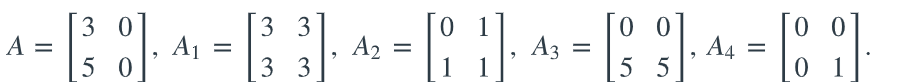# Find the coordinate vector of A relativeFatoom Alsaadi 2022-03-31 Answered

Find the coordinate vector of A relative to the basis S = {A 1, A 2, A 3, A 4 }.(A)S = (_,_,_,_)

You can still ask an expert for help

• Questions are typically answered in as fast as 30 minutes

Solve your problem for the price of one coffee

• Math expert for every subject
• Pay only if we can solve ituser_27qwe

$\left[\begin{array}{cc}3& 0\\ 5& 0\end{array}\right]+\left[\begin{array}{cc}3& 3\\ 3& 3\end{array}\right]+\left[\begin{array}{cc}0& 1\\ 1& 1\end{array}\right]+\left[\begin{array}{cc}0& 0\\ 5& 5\end{array}\right]+\left[\begin{array}{cc}0& 0\\ 0& 1\end{array}\right]$

$\left[\begin{array}{cc}3+3& 0+3\\ 5+3& 0+3\end{array}\right]+\left[\begin{array}{cc}0& 1\\ 1& 1\end{array}\right]+\left[\begin{array}{cc}0& 0\\ 5& 5\end{array}\right]+\left[\begin{array}{cc}0& 0\\ 0& 1\end{array}\right]$

Simplify each element of the matrix $\left[\begin{array}{cc}3+3& 0+3\\ 5+3& 0+3\end{array}\right]$.

Simplify $3+3$.

$\left[\begin{array}{cc}6& 0+3\\ 5+3& 0+3\end{array}\right]+\left[\begin{array}{cc}0& 1\\ 1& 1\end{array}\right]+\left[\begin{array}{cc}0& 0\\ 5& 5\end{array}\right]+\left[\begin{array}{cc}0& 0\\ 0& 1\end{array}\right]$

Simplify $0+3$.

$\left[\begin{array}{cc}6& 3\\ 5+3& 0+3\end{array}\right]+\left[\begin{array}{cc}0& 1\\ 1& 1\end{array}\right]+\left[\begin{array}{cc}0& 0\\ 5& 5\end{array}\right]+\left[\begin{array}{cc}0& 0\\ 0& 1\end{array}\right]$

Simplify $5+3$.

$\left[\begin{array}{cc}6& 3\\ 8& 0+3\end{array}\right]+\left[\begin{array}{cc}0& 1\\ 1& 1\end{array}\right]+\left[\begin{array}{cc}0& 0\\ 5& 5\end{array}\right]+\left[\begin{array}{cc}0& 0\\ 0& 1\end{array}\right]$

Simplify $0+3$.

$\left[\begin{array}{cc}6& 3\\ 8& 3\end{array}\right]+\left[\begin{array}{cc}0& 1\\ 1& 1\end{array}\right]+\left[\begin{array}{cc}0& 0\\ 5& 5\end{array}\right]+\left[\begin{array}{cc}0& 0\\ 0& 1\end{array}\right]$

$\left[\begin{array}{cc}6+0& 3+1\\ 8+1& 3+1\end{array}\right]+\left[\begin{array}{cc}0& 0\\ 5& 5\end{array}\right]+\left[\begin{array}{cc}0& 0\\ 0& 1\end{array}\right]$

Simplify each element of the matrix $\left[\begin{array}{cc}6+0& 3+1\\ 8+1& 3+1\end{array}\right]$.

Simplify $6+0$.

$\left[\begin{array}{cc}6& 3+1\\ 8+1& 3+1\end{array}\right]+\left[\begin{array}{cc}0& 0\\ 5& 5\end{array}\right]+\left[\begin{array}{cc}0& 0\\ 0& 1\end{array}\right]$

Simplify $3+1$.

$\left[\begin{array}{cc}6& 4\\ 8+1& 3+1\end{array}\right]+\left[\begin{array}{cc}0& 0\\ 5& 5\end{array}\right]+\left[\begin{array}{cc}0& 0\\ 0& 1\end{array}\right]$

Simplify $8+1$.

$\left[\begin{array}{cc}6& 4\\ 9& 3+1\end{array}\right]+\left[\begin{array}{cc}0& 0\\ 5& 5\end{array}\right]+\left[\begin{array}{cc}0& 0\\ 0& 1\end{array}\right]$

Simplify $3+1$.

$\left[\begin{array}{cc}6& 4\\ 9& 4\end{array}\right]+\left[\begin{array}{cc}0& 0\\ 5& 5\end{array}\right]+\left[\begin{array}{cc}0& 0\\ 0& 1\end{array}\right]$

$\left[\begin{array}{cc}6+0& 4+0\\ 9+5& 4+5\end{array}\right]+\left[\begin{array}{cc}0& 0\\ 0& 1\end{array}\right]$

Simplify each element of the matrix $\left[\begin{array}{cc}6+0& 4+0\\ 9+5& 4+5\end{array}\right]$.

Simplify $6+0$.

$\left[\begin{array}{cc}6& 4+0\\ 9+5& 4+5\end{array}\right]+\left[\begin{array}{cc}0& 0\\ 0& 1\end{array}\right]$

Simplify $4+0$.

$\left[\begin{array}{cc}6& 4\\ 9+5& 4+5\end{array}\right]+\left[\begin{array}{cc}0& 0\\ 0& 1\end{array}\right]$

Simplify $9+5$.

$\left[\begin{array}{cc}6& 4\\ 14& 4+5\end{array}\right]+\left[\begin{array}{cc}0& 0\\ 0& 1\end{array}\right]$

Simplify $4+5$.

$\left[\begin{array}{cc}6& 4\\ 14& 9\end{array}\right]+\left[\begin{array}{cc}0& 0\\ 0& 1\end{array}\right]$

$\left[\begin{array}{cc}6+0& 4+0\\ 14+0& 9+1\end{array}\right]$

Simplify each element of the matrix $\left[\begin{array}{cc}6+0& 4+0\\ 14+0& 9+1\end{array}\right]$.

Simplify $6+0$.

$\left[\begin{array}{cc}6& 4+0\\ 14+0& 9+1\end{array}\right]$

Simplify $4+0$.

$\left[\begin{array}{cc}6& 4\\ 14+0& 9+1\end{array}\right]$

Simplify $14+0$.

$\left[\begin{array}{cc}6& 4\\ 14& 9+1\end{array}\right]$

Simplify $9+1$.

$\left[\begin{array}{cc}6& 4\\ 14& 10\end{array}\right]$ - Answer